## Example Questions

### Example Question #5 : Negative Numbers

If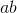is a positive number, and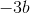is also a positive number, what is a possible value for?Explanation:

Because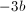is positive,must be negative since the product of two negative numbers is positive.

Because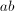is also positive,must also be negative in order to produce a prositive product.

To check you answer, you can try plugging in any negative number for.

### Example Question #11 : Concepts

Multiply the following negative numbers: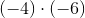.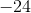Explanation:

When two negative numbers are multiplied by each other, the result is a positive number.

When a negative and a nonnegative number are multiplied with each other, the result is a negative number.

Therefore, sinceandare negative numbers, the product must be positive.

Therefore, the answer is positive.

### Example Question #1 : How To Multiply Negative Numbers

Multiply: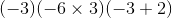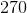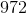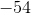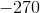Explanation:

Simplify the expression term by term.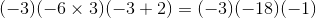A negative number multiplied by a negative number gives a positive number.

A negative number multiplied by a positive number gives a negative number.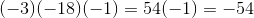### Example Question #2 : How To Multiply Negative Numbers

Multiply: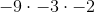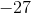Explanation:

Multiply the first two terms.  A negative number times another negative will result in a positive number.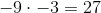Multiply 27 with negative 2.  A positive number times a negative number will result in a negative number.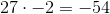The answer is:### Example Question #3 : How To Multiply Negative Numbers

Solve the following equation: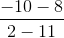Explanation: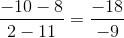If there are two negative signs, then the answer will be positive. If there were only one negative sign, the answer will be negative.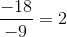### Example Question #4 : How To Multiply Negative Numbers

Simplify the following expression: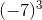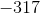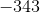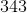Explanation:

Simplify the following expression:Let's begin by expanding the inside of the parentheses.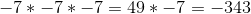Now, it is important to use all three negative signs. The first two will cancel out, but the third one will remain, ensuring our final answer will be negative.